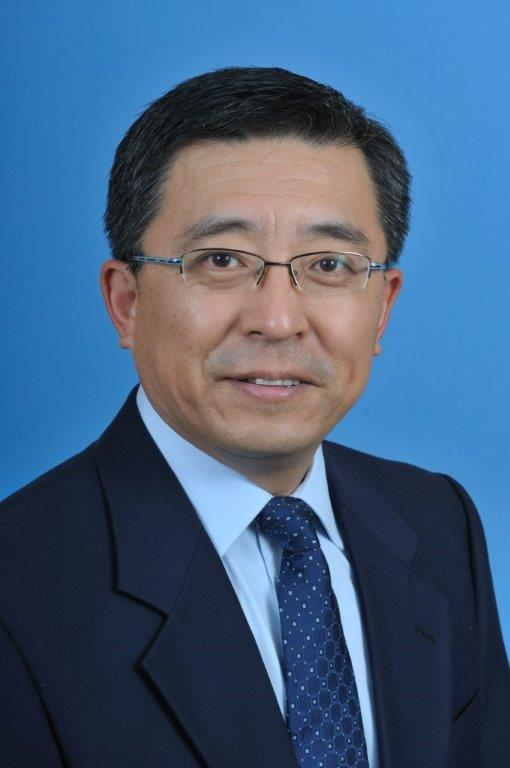# Dr. Wu JingProfessor in Mathematics

Office: Science & Technology Building 418

Phone: (910) 672-2205

Email: wjing@uncfsu.edu

Personal Homepage: https://fsusites.uncfsu.edu/wjing/

BIOGRAPHY

Dr. Wu Jing is a professor of mathematics in the Department of Mathematics & Computer Science at Fayetteville State University. He joined Fayetteville State University in 2006 as an assistant professor.

Dr. Jing's research areas include applied Harmonic analysis, operator algebras, operator theory, preserver problems, mappings on algebras and rings, and quantum logics. He is an active reviewer for both Mathematical Reviews and Zentralblatt MATH. Dr. Jing has been served as referee for about thirty international journals. Dr. Jing also has great interest in K-12 math competitions, such as MATHCOUNTS and AMC8/10/12.

TEACHING

MATH 121 Introduction to College Algebra
MATH 123 College Algebra
MATH 129 Pre-calculus Mathematics I
MATH 130 Pre-calculus Mathematics II
MATH 140 Applied Calculus
MATH 142 Calculus with Analytic Geometry I
MATH 150 Discrete Mathematics I
MATH 241 Calculus with Analytic Geometry II
MATH 431 Differential Equations II
MATH 507 Linear Algebra

RESEARCH INTERESTS

Applied Harmonic Analysis
Functional Analysis
Operator Algebra
Maps on Algebras & Rings
Preserver Problems
Quantum Logic

SELECTED PUBLICATIONS

(with S. Ali and A. Fošner) On Generalized Derivations and Centralizers of Operator Algebras with Involution, J. Contemp. Math. Anal. 53 (2018), 27-33.

Nonlinear *-Lie derivations of standard operator algebras, Quaestiones Math. 39 (2016), 1037-1046.

(with D. Han, D. Larson, P. Li, and R. Mohapatra) Dilation of dual frame pairs in Hilbert C*-modules, Results Math. 63 (2013), 241-250.

(with F. Lu) Additivity of Jordan (triple) derivations on rings, Comm. Algebra 40 (2012), 2700-2719.

(with F. Lu) Lie derivable mappings on prime rings, Linear Multilinear Algebra 60 (2012), 167-180.

(with F. Lu) Characterizations of Lie derivations of B(X)Linear Algebra Appl.  432 (2010), 89-99.

(with D. Han and R. Mohapatra) Perturbation of frames and Riesz bases in Hilbert C*-modules,  Linear Algebra Appl. 431 (2009), 746-759.

On Jordan all-derivable points of B(H)Linear Algebra Appl.  430 (2009), 941-946.

(with X. Cheng) Additivity of maps on triangular algebras, Electron. J. Linear Algebra  17 (2008), 597-615.

(with D. Han, D. Larson, and R.  Mohapatra) Riesz bases and their dual modular frames in Hilbert C*-modules, J. Math. Anal. Appl.  343 (2008), 246-256.

(with D. Han and R. Mohapatra) Structured Parseval frames in Hilbert C*-modules, Contemp. Math. 414 (2006), 275-287.

(with P. Li and J. Ma) Additive derivations of certain reflexive algebras, Houston J. Math.  32 (2006), 521-530.

Ideals, filters, and supports in pseudoeffect algebras, Internat. J. Theoret. Phys. 43 (2004), 349-358.

(with P. Li) Jordan elementary maps on rings, Linear Algebra Appl. 382 (2004), 237-245.

(with S. Lu) Generalized Jordan derivations on prime rings and standard operator algebras, Taiwanese J. Math. (2003), 605-613.

(with S. Lu) Topological reflexivity of the spaces of (α, β)-derivations on operator algebras, Studia Math. 156 (2003), 121-131.

(with P. Li and S. Lu) Additive mappings that preserve rank one nilpotent operators,Linear  Algebra Appl. 367 (2003), 213-224.

(with P. Li and S. Lu) Characterizations of derivations on some operator algebras,Bull. Austral. Math. Soc. 66 (2002), 227-232.

(with P. Li and S. Lu) Ring automorphisms of reflexive algebras,  Chinese Ann. Math. Ser. A 23 (2002), 41-48.

(with X. Wang) Bilocal derivations of reflexive algebras, J. Math. Res. Exposition 21 (2001), 197-202.

Local derivations of reflexive algebras II, Proc. Amer. Math. Soc. 129 (2001), 1733-1737.

Additive mappings preserving zero products, Acta Math. Sinica  42 (1999), 1125-1128.

Spectrum-preserving multiplicative maps, Acta Math. Sinica (N.S.) 14 (1998), 719-722.

Local derivations of reflexive algebras,  Proc. Amer. Math. Soc. 125 (1997), 869-873.